Ex 1.1

Chapter 1 Class 12 Relation and Functions
Serial order wise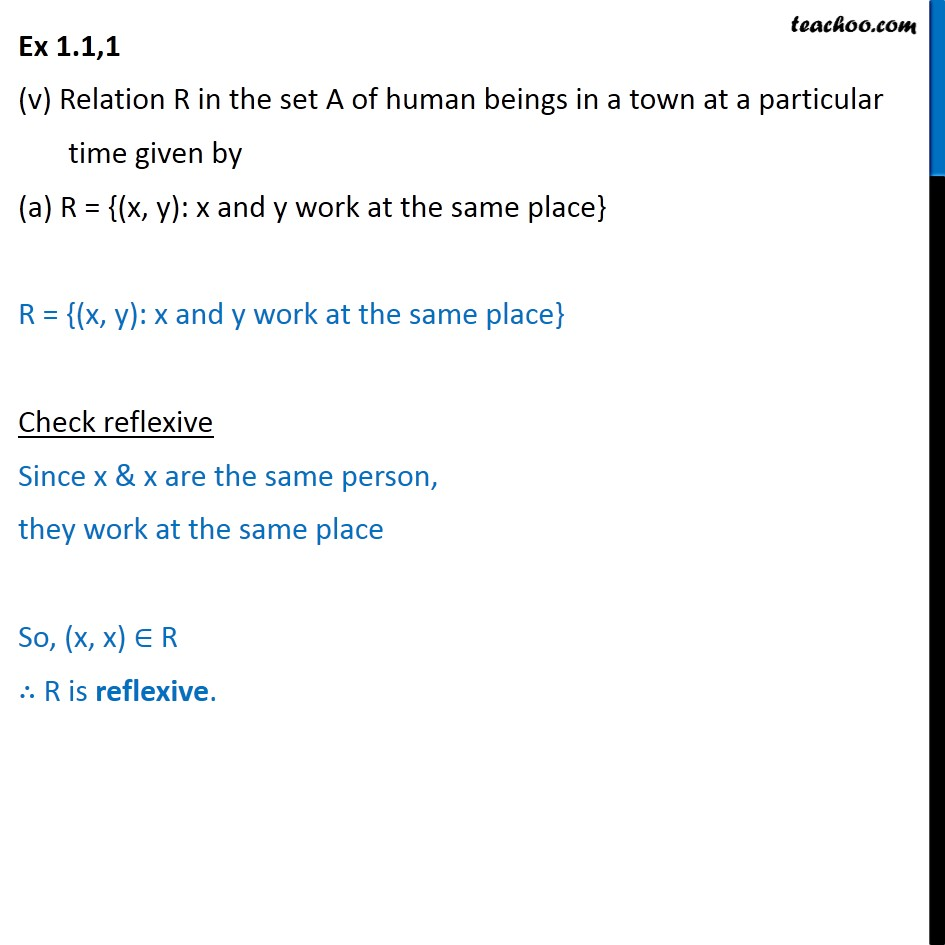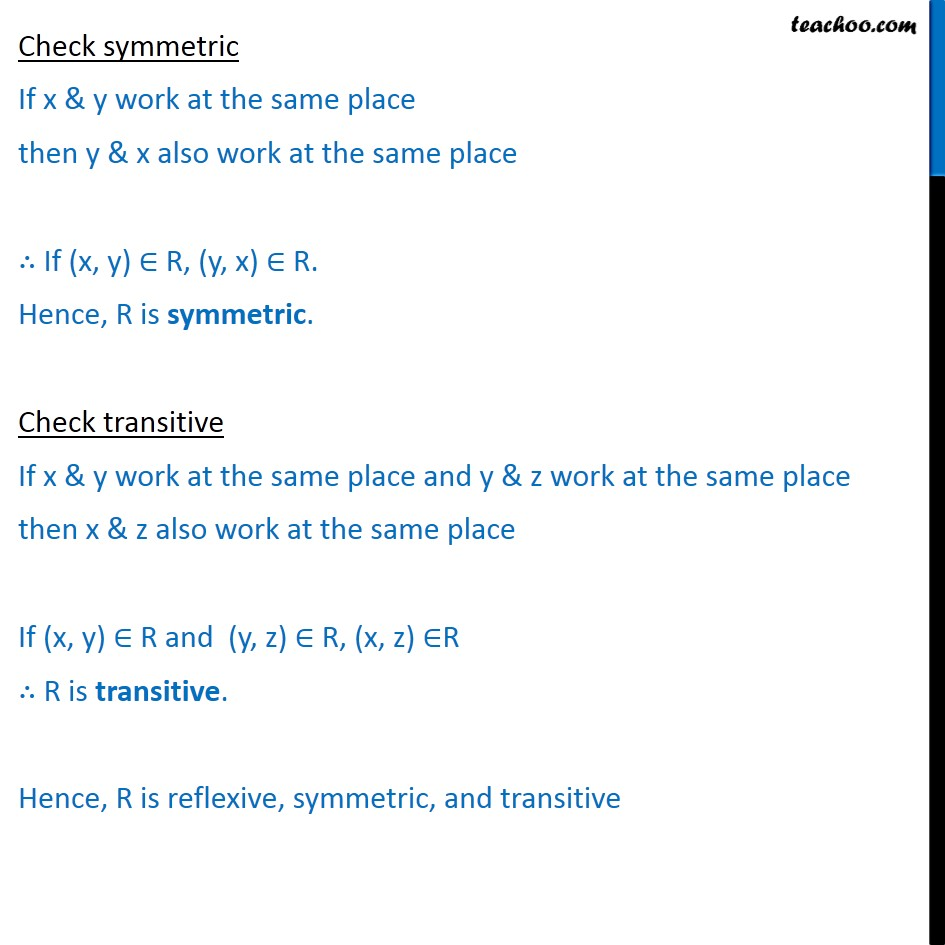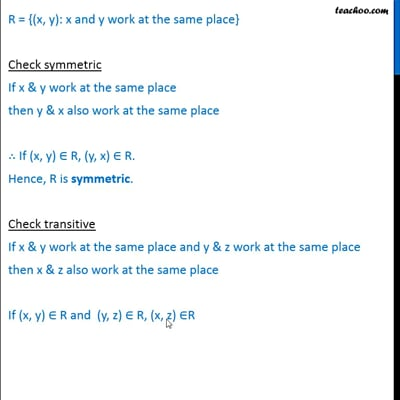This video is only available for Teachoo black users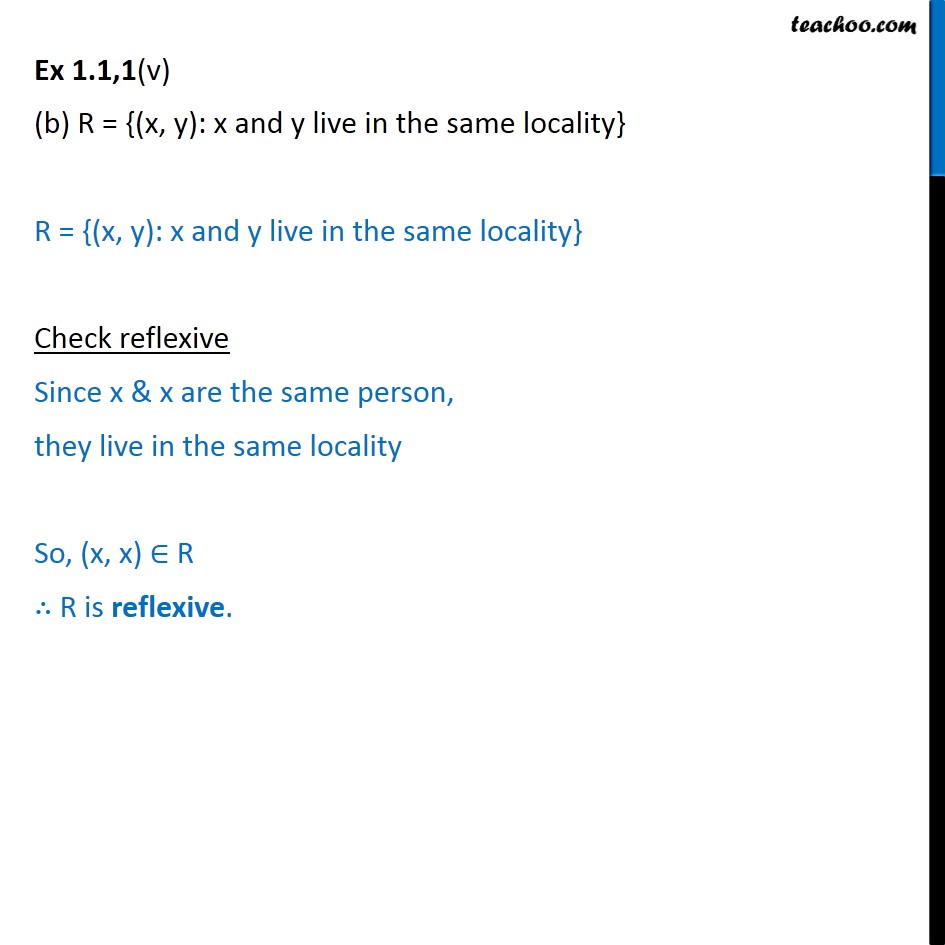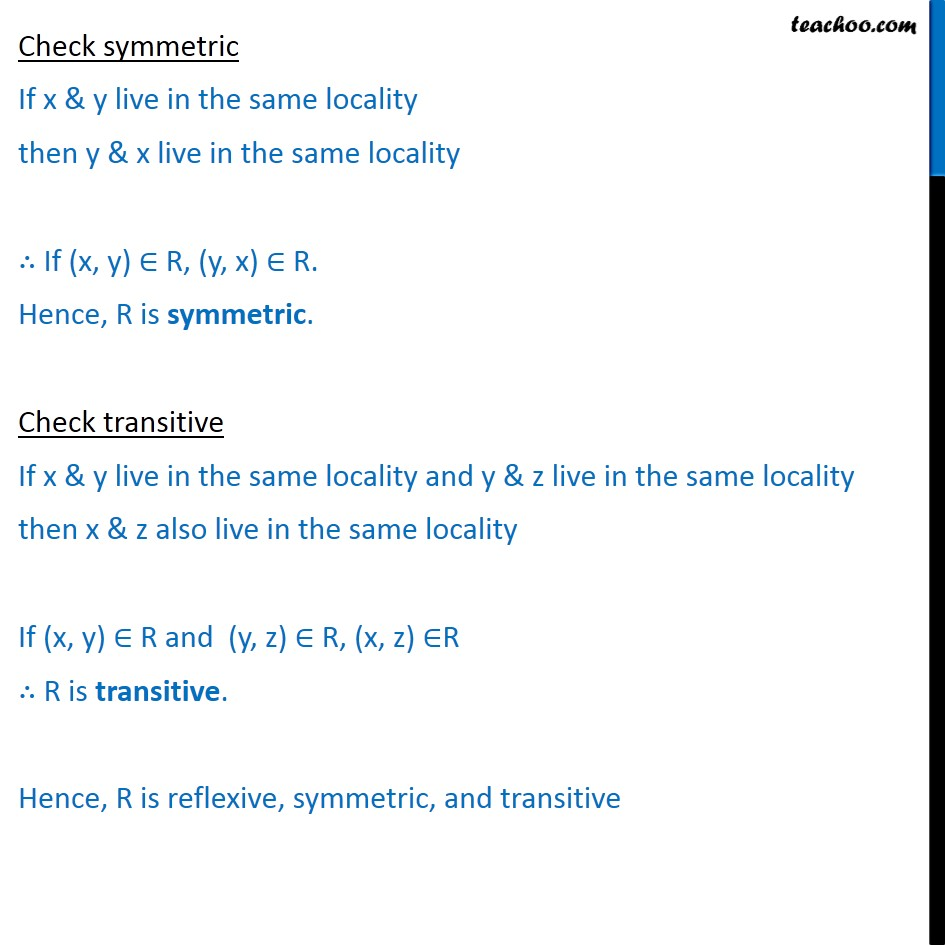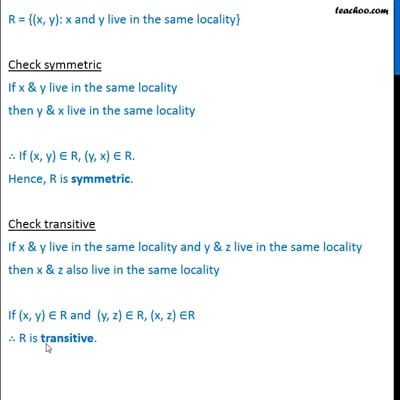This video is only available for Teachoo black users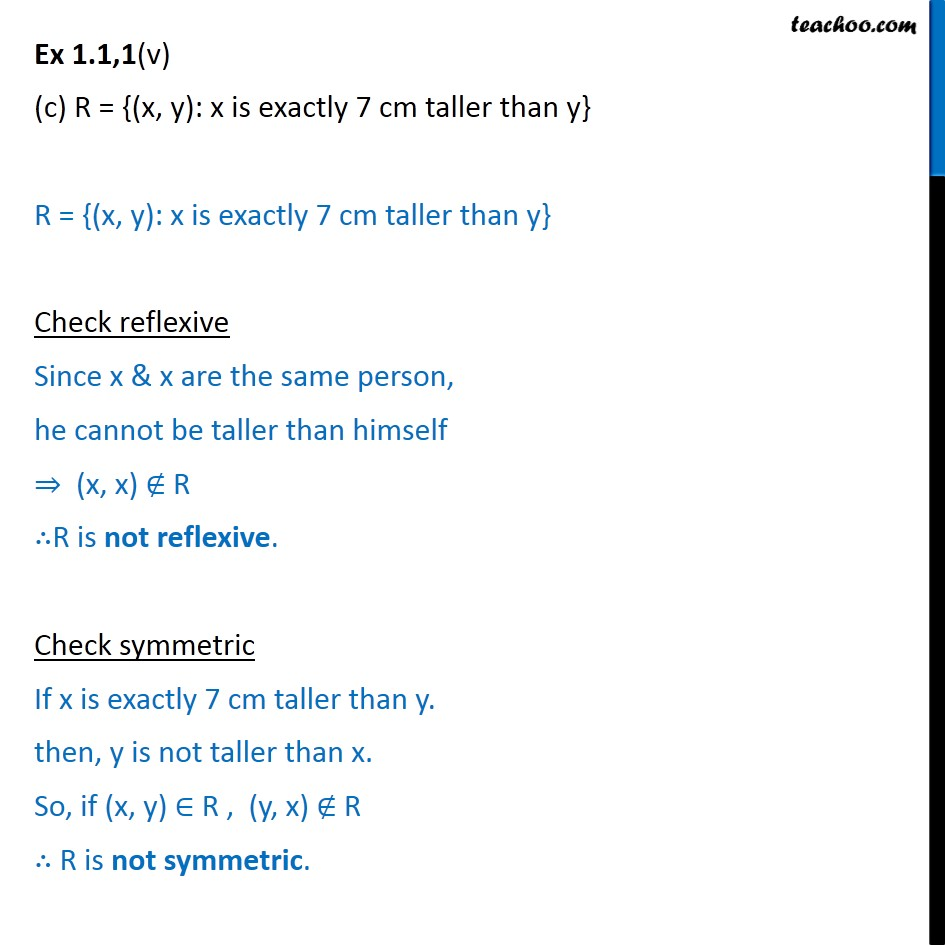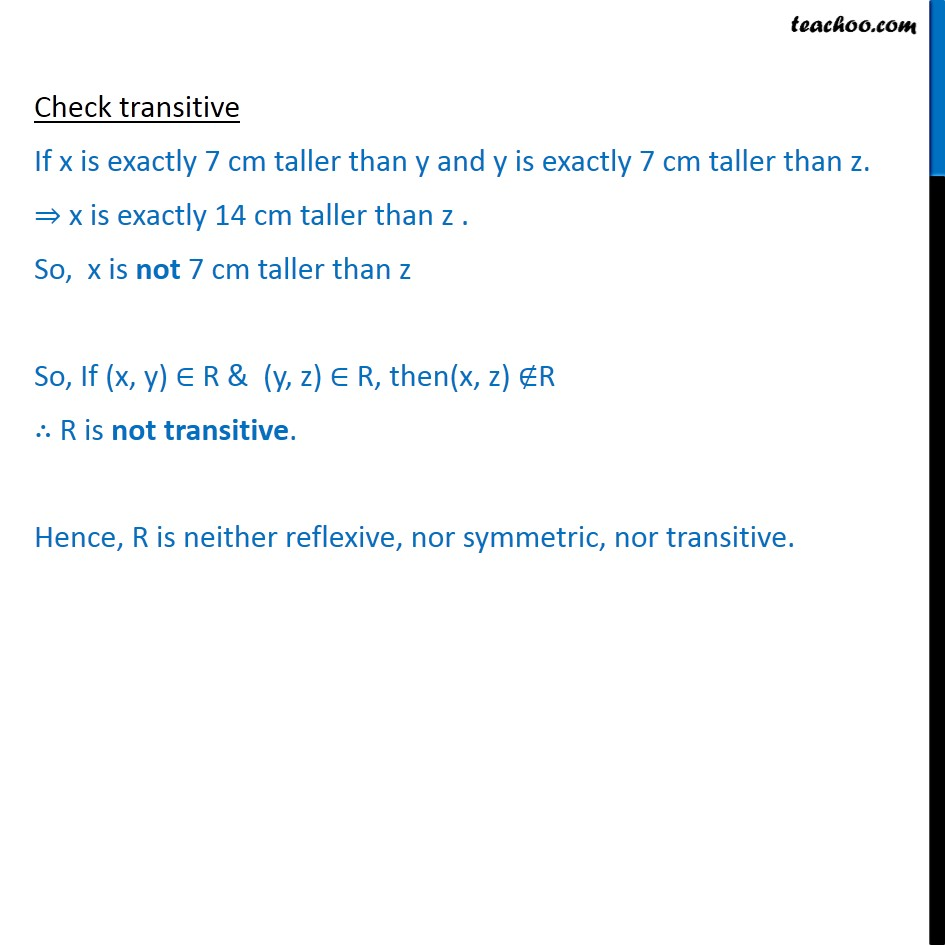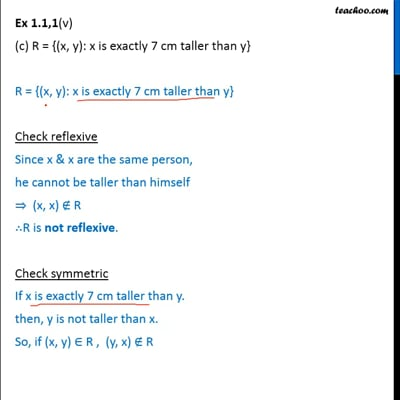This video is only available for Teachoo black users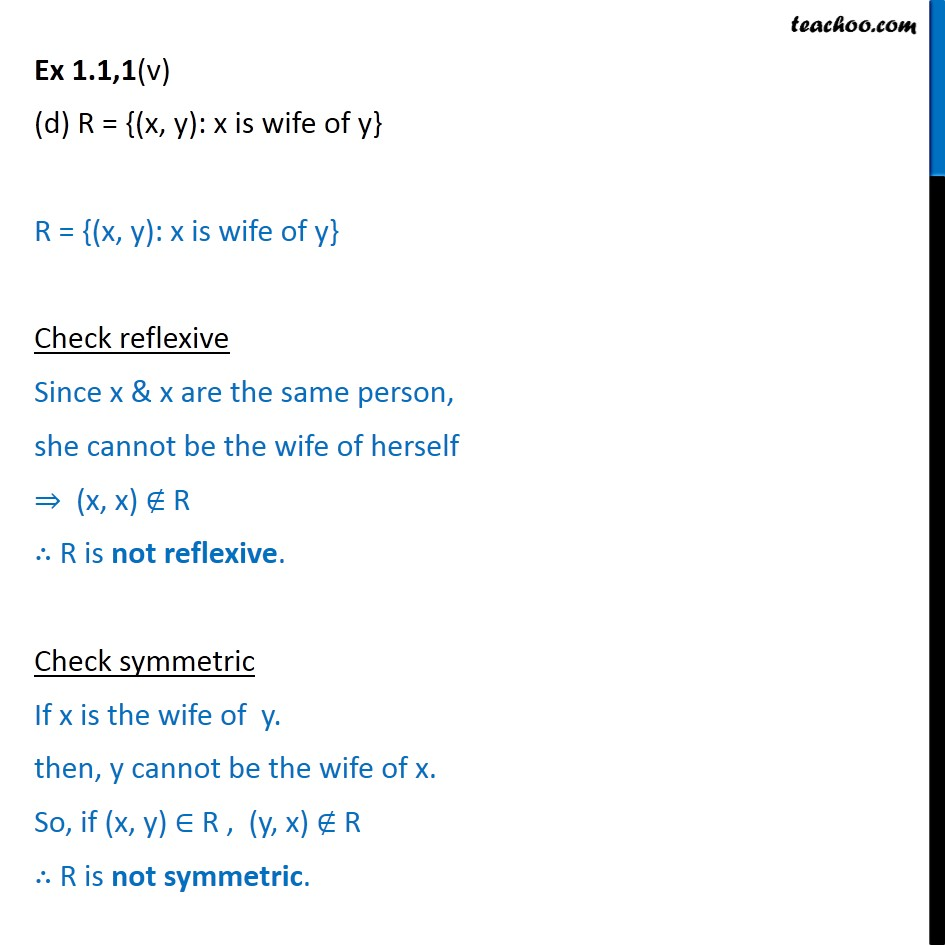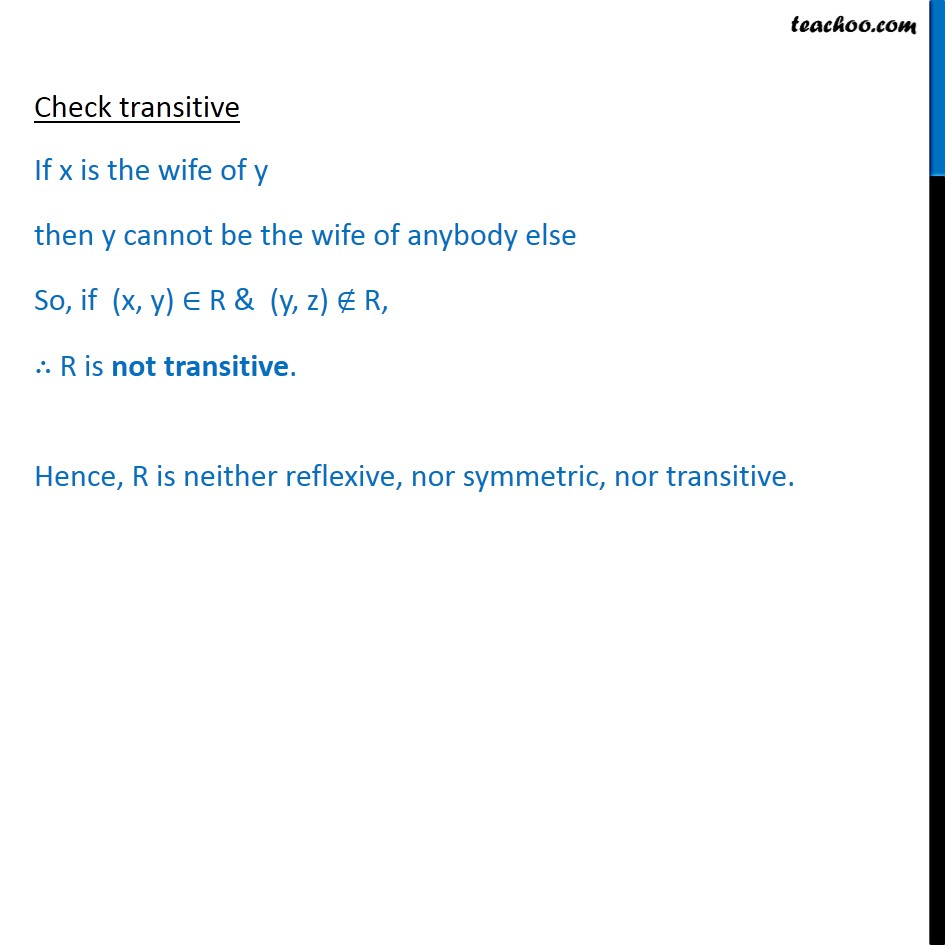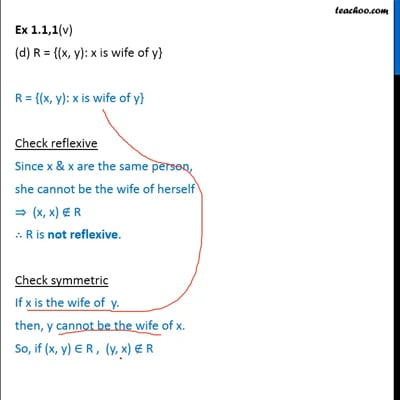This video is only available for Teachoo black users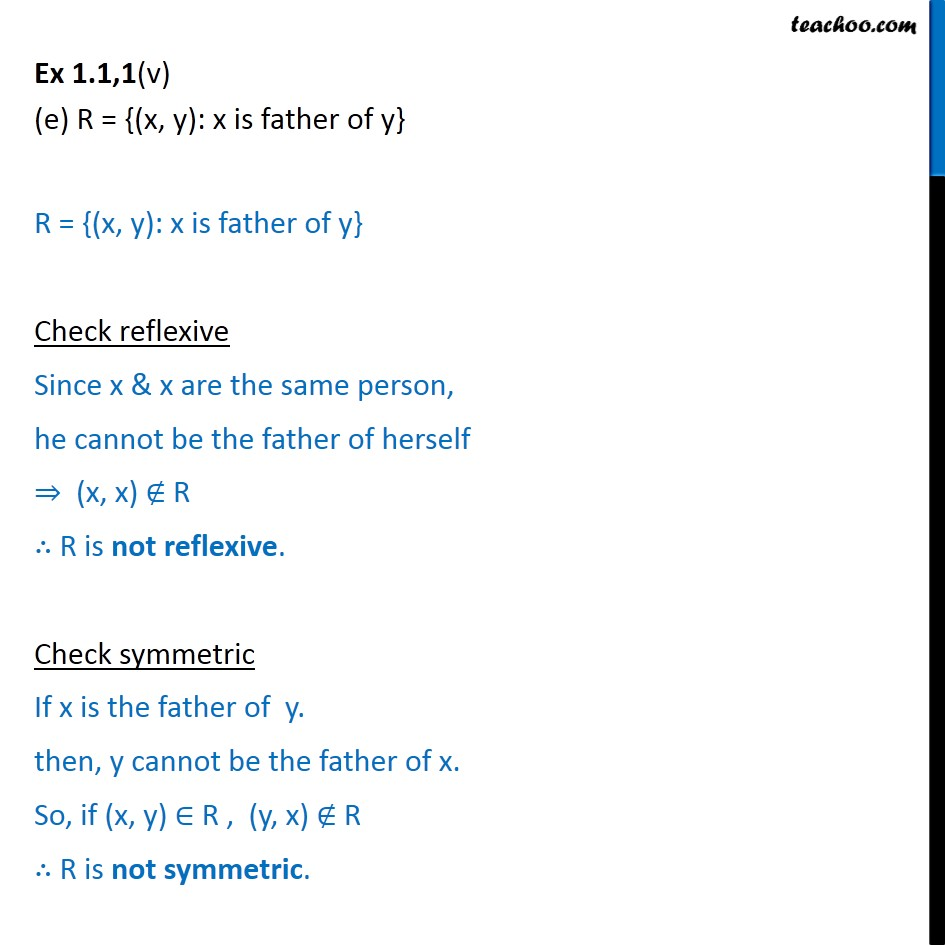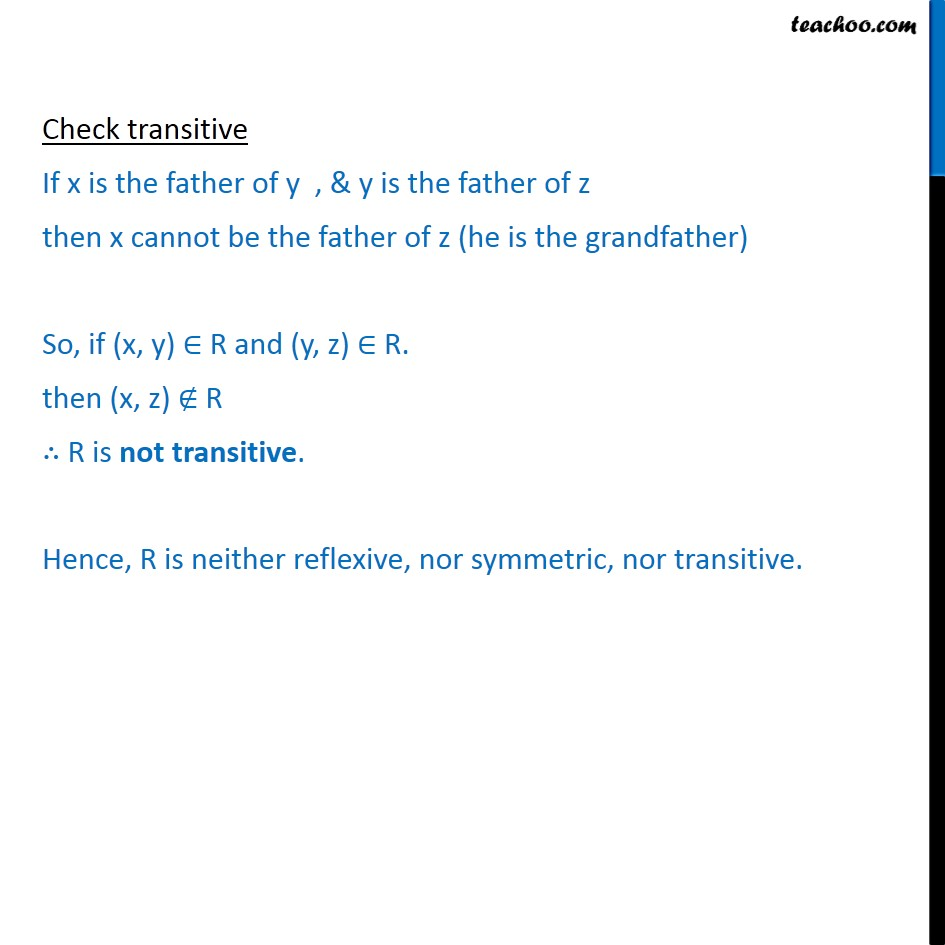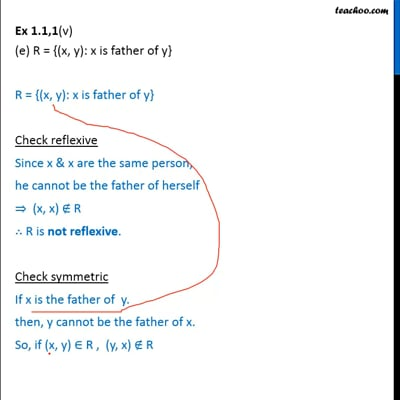This video is only available for Teachoo black users

Maths Crash Course - Live lectures + all videos + Real time Doubt solving!

### Transcript

Ex 1.1,1 (v) Relation R in the set A of human beings in a town at a particular time given by (a) R = {(x, y): x and y work at the same place} R = {(x, y): x and y work at the same place} Check reflexive Since x & x are the same person, they work at the same place So, (x, x) R R is reflexive. Check symmetric If x & y work at the same place then y & x also work at the same place If (x, y) R, (y, x) R. Hence, R is symmetric. Check transitive If x & y work at the same place and y & z work at the same place then x & z also work at the same place If (x, y) R and (y, z) R, (x, z) R R is transitive. Hence, R is reflexive, symmetric, and transitive Ex 1.1,1(v) (b) R = {(x, y): x and y live in the same locality} R = {(x, y): x and y live in the same locality} Check reflexive Since x & x are the same person, they live in the same locality So, (x, x) R R is reflexive. Check symmetric If x & y live in the same locality then y & x live in the same locality If (x, y) R, (y, x) R. Hence, R is symmetric. Check transitive If x & y live in the same locality and y & z live in the same locality then x & z also live in the same locality If (x, y) R and (y, z) R, (x, z) R R is transitive. Hence, R is reflexive, symmetric, and transitive Ex 1.1,1(v) (c) R = {(x, y): x is exactly 7 cm taller than y} R = {(x, y): x is exactly 7 cm taller than y} Check reflexive Since x & x are the same person, he cannot be taller than himself (x, x) R R is not reflexive. Check symmetric If x is exactly 7 cm taller than y. then, y is not taller than x. So, if (x, y) R , (y, x) R R is not symmetric. Check transitive If x is exactly 7 cm taller than y and y is exactly 7 cm taller than z. x is exactly 14 cm taller than z . So, x is not 7 cm taller than z So, If (x, y) R & (y, z) R, then(x, z) R R is not transitive. Hence, R is neither reflexive, nor symmetric, nor transitive. Ex 1.1,1(v) (d) R = {(x, y): x is wife of y} R = {(x, y): x is wife of y} Check reflexive Since x & x are the same person, she cannot be the wife of herself (x, x) R R is not reflexive. Check symmetric If x is the wife of y. then, y cannot be the wife of x. So, if (x, y) R , (y, x) R R is not symmetric. Check transitive If x is the wife of y then y cannot be the wife of anybody else So, if (x, y) R & (y, z) R, R is not transitive. Hence, R is neither reflexive, nor symmetric, nor transitive. Ex 1.1,1(v) (e) R = {(x, y): x is father of y} R = {(x, y): x is father of y} Check reflexive Since x & x are the same person, he cannot be the father of herself (x, x) R R is not reflexive. Check symmetric If x is the father of y. then, y cannot be the father of x. So, if (x, y) R , (y, x) R R is not symmetric. Check transitive If x is the father of y , & y is the father of z then x cannot be the father of z (he is the grandfather) So, if (x, y) R and (y, z) R. then (x, z) R R is not transitive. Hence, R is neither reflexive, nor symmetric, nor transitive.